# C Program to Check Whether Number is Perfect Or Not

In this tutorial you will learn about the C Program to Check Whether Number is Perfect Or Not and its application with practical example.

In this tutorial, we will learn to create a C program that will find Perfect Number using C programming.

## Prerequisites

Before starting with this tutorial we assume that you are best aware of the following C programming topics:

• C Operators.
• Loop statements.
• For Loop.
• Basic input/output.

## What Is Perfect Number?

Perfect number is a positive integer number,that equals to sum of its positive divisors not include the number itself is equal to that number.

## Example

Divisor of  6 are 1,2 and 3. and sum of these  are (1+2+3=6). So we can say that 6 is a perfect number in which we will not induced 6 i.e exclude 6 .So number is  called perfect when divisor of number added and that create the number itself called Perfect number.

## C Program to Check Whether Number is Perfect Or Not

So here we are going to create a program in which we take a value from user and we will find the given number is perfect or not? By method using adding all divisors of a number.

## Output

Perfect number.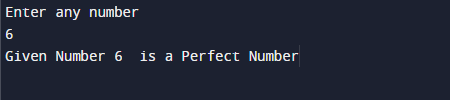Not a perfect number.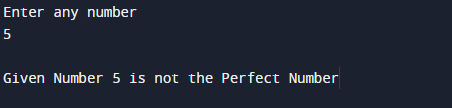In the above program, we have first declared and initialized a set variables required in the program.

• no   =  holding given number
• i       = for iteration of a loop.

Explanation of program, First we take the integer value in ‘no’ variable. So the condition is that A Perfect number is a number which is equal to sum of its divisor.

Example, 6’s divisor  are 1, 2 and 3. and there sum is (1+2+3=6) ,the divisors of  6. So the number 6 is called as perfect number.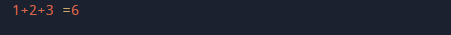From the above image of a perfect number in c,Suppose  user entered value is: no = 6
Step by step explanation for loop is here.

In 1st Iteration…
Number is no= 6, sum = 0 and loop start at i = 1.
If (no % i == 0)  // if( 6%1== 0 ) true.
here number is complete divisible So,
sum = sum + i  // sum= 0 + 1 =1.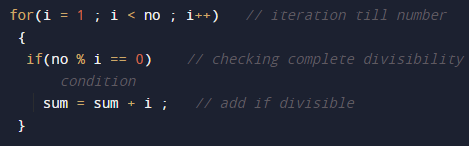Now,Second Iteration..
Values of  sum and i has altered as sum = 1 and i = 2.

If (no % i == 0) // if( 6 %2 ==0 ) true.
sum = sum + i // sum = 1 + 2 = 3.

Third Iteration.
Again the values of both sum and i has altered as sum = 3 and i = 3

If (6 % 3 == 0)  // if( 6%3==0) true.
sum = sum + i //sum = 3 + 3 = 6.

Next fourth and fifth iterations ,condition inside the if will fail
6 % 4 == 0 (FALSE)
6 % 5 == 0 (FALSE)

In next iteration, the value of i becomes 6, So (6 < 6) false . So, the compiler will terminate the for loop.
Then, we check whether the value of sum  is exactly equal to the no or Not.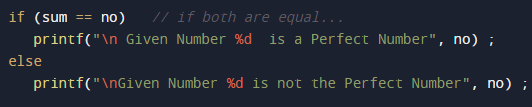If it is TRUE, the below print f statement will execute. else print f statement will execute.

In this tutorial we have learn about the C Program to Check Whether Number is Perfect Or Not and its application with practical example. I hope you will like this tutorial.